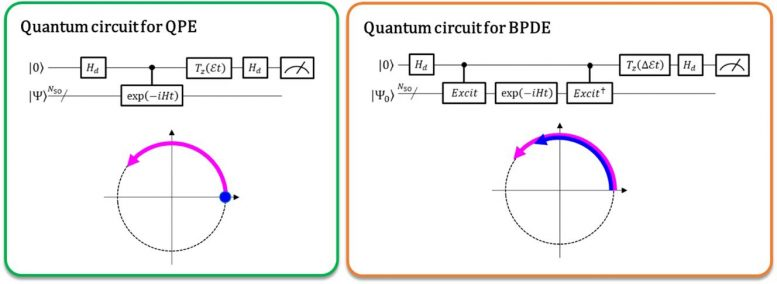# New Quantum Algorithm Directly Calculates the Energy Difference of Atoms and MoleculesLeft: The phase difference between |0⟩|Ψ⟩ and exp(-iEt)|1⟩|Ψ⟩ affords the total energy E . The curved arrow in purple indicates the phase evolution of |Ψ⟩ in time. Right: The phase difference between exp(-iE0t)|0⟩|Ψ0 ⟩ and exp(-iE1t)|1⟩|Ψ1 ⟩ affords the energy difference E1 – E0, directly. The curved arrows in blue and in purple indicate the phase evolution of |Ψ0 ⟩ and that of |Ψ1 ⟩, respectively. Credit: K. Sugisaki, K. Sato and T. Takui

Osaka City University creates a general quantum algorithm, executable on quantum computers, which calculates molecular energy differences without considering relevant total energies.

As newly reported by the journal Physical Chemistry Chemical Physics, researchers from the Graduate School of Science at Osaka City University have developed a quantum algorithm that can understand the electronic states of atomic or molecular systems by directly calculating the energy difference in their relevant states. Implemented as a Bayesian phase different estimation, the algorithm breaks from convention by not focusing on the difference in total energies calculated from the pre- and post-phase evolution, but by following the evolution of the energy difference itself.

“Almost all chemistry problems discuss the energy difference, not the total energy of the molecule itself,” says research lead and Specially-Appointed Lecturer Kenji Sugisaki, “also, molecules with heavy atoms that appear at the lower part of the periodic table have large total energies, but the size of the energy difference discussed in chemistry, such as electronic excitation states and ionization energies, does not depend much on the size of the molecule.” This idea led Sugisaki and his team to implementing a quantum algorithm that directly calculates energy differences instead of total energies, creating a future where scalable or practical quantum computers enable us to carry out actual chemical research and materials development.

Currently, quantum computers are capable of performing the full configuration interaction (full-CI) calculations which afford optimal molecular energies with a quantum algorithm called quantum phase estimation (QPE), noting that the full-CI calculation for sizable molecular systems is intractable with any supercomputers. QPE relies on the fact that a wave function, |Ψ⟩ which denotes the mathematical description of the quantum state of a microscopic system – in this case the mathematical solution of the Schrödinger equation for the microscopic system such as an atom or molecule – time-evolutionally changes its phase depending on its total energy. In the conventional QPE, the quantum superposition state (|0⟩|Ψ⟩+|1⟩|Ψ⟩) ⁄ √2 is prepared, and the introduction of a controlled time evolution operator makes |Ψ⟩ evolve in time only when the first qubit designates the |1⟩ state. Thus, the |1⟩ state creates a quantum phase of the post-evolution in time whereas the|0⟩ state that of the pre-evolution. The phase difference between the pre- and post-evolutions gives the total energy of the system.

The researchers of Osaka City University generalize the conventional QPE to the direct calculation of the difference in the total energy between two relevant quantum states. In the newly implemented quantum algorithm termed Bayesian phase difference estimation (BPDE), the superposition of the two wave functions, (|0⟩|Ψ0 ⟩ + |1⟩|Ψ1 ⟩) ⁄ √2, where |Ψ0 ⟩ and |Ψ1 ⟩ denote the wave function relevant to each state, respectively, is prepared, and the difference in the phase between |Ψ0 ⟩ and |Ψ1 ⟩ after the time evolution of the superposition directly gives the difference in the total energy between the two wave functions involved. “We emphasize that the algorithm follows the evolution of the energy difference over time, which is less prone to noise than individually calculating the total energy of an atom or molecule. Thus, the algorithm suites the need for chemistry problems which require precise accuracy in energy.” states research supervisor and Professor Emeritus Takeji Takui.

Previously, this research group developed a quantum algorithm that directly calculates the energy difference between electronic states (spin states) with different spin quantum numbers (K. Sugisaki, K. Toyota, K. Sato, D. Shiomi, T. Takui, Chem. Sci. 2021, 12, 2121–2132.). This algorithm, however, requires more qubits than the conventional QPE and cannot be applied to the energy difference calculation between the electronic states with equal spin quantum numbers, which is important for the spectral assignment of UV-visible absorption spectra. The BPDE algorithm developed in the study overcomes these issues, making it a highly versatile quantum algorithm.

Reference: “A Bayesian phase difference estimation: a general quantum algorithm for the direct calculation of energy gaps” 2 September 2021, Physical Chemistry Chemical Physics.

Other contributors include Kazuo Toyota, Kazunobu Sato and Daisuke Shiomi, all of whom are affiliated with the Department of Chemistry and Molecular Materials Science in Osaka City University’s Graduate School of Science. Sugisaki is also affiliated with the Japan Science and Technology Agency’s PRESTO Project, “Quantum Software.” Takui is also a University Research Administrator in the Research Support Department/University Research Administrator Center of Osaka City University.

#### 3 Commentson "New Quantum Algorithm Directly Calculates the Energy Difference of Atoms and Molecules"

1.BibhutibhusanPatel | September 2, 2021 at 11:01 pm | Reply

The QPE quantum algorithm ìs correspondent to wave-particle dual proporties of photon.

2.BibhutibhusanPatel | September 2, 2021 at 11:51 pm | Reply

The QPE can show photon having dùal pròporties of wave-particle.Here in superposition single point is considered for two phases post- and pre-,ĺìkely the total basic background energy evolves as self ìs the difference of energies between two phases.

3.BibhutibhusanPatel | September 2, 2021 at 11:58 pm | Reply

The QPE can show photon having dùal pròporties of wave-particle.Here in superposition single point is considered for two phases post- and pre-,ĺìkely the total basic background energy evolves as self ìs the difference of energies between two phases.The present ĺaw showing wave-partical nature of photon is confirming from other contemporary reseach resuĺts on QC.